## IceMan Reference Guide

#### Compositing

The basic image merging operations defined by Porter and Duff in their 1984 paper, with a couple of additions.  All these operations are methods of Image and return a new Image instance.

Each of the following operations is illustrated with these foreground and background images: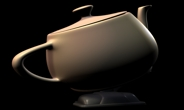teapot.tifAlpha channel of "teapot.tif"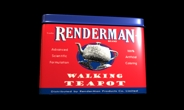tinbox.tif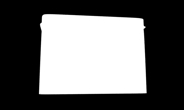Alpha channel of "tinbox.tif"
Over(fg)
fg: Foreground image (Image)

The image being operated on is the background image.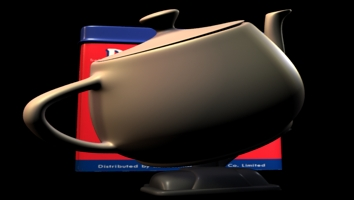``` fg := IceImage With("teapot.tif") bg := IceImage With("tinbox.tif") result := bg Over(fg) ```
Cover(fg)
fg: Foreground image

Correlated Over operation.  Similar to Over, but works better for correlated subpixel coverage.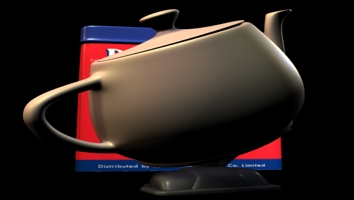``` fg := IceImage With("teapot.tif") bg := IceImage With("tinbox.tif") result := bg Cover(fg) ```
Atop(fg)
fg: Foreground image (Image)

Merge "atop".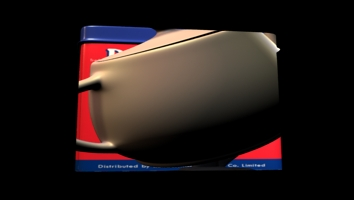``` fg := IceImage With("teapot.tif") bg := IceImage With("tinbox.tif") result := bg Atop(fg) ```
In(fg)
fg: Foreground image (Image)

Merge "in".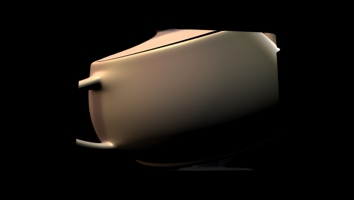``` fg := IceImage with('teapot.tif') bg := IceImage with('tinbox.tif') result := bg In(fg) ```
MergeXor(fg)
fg: Foreground image (Image)

Merge XOR operation.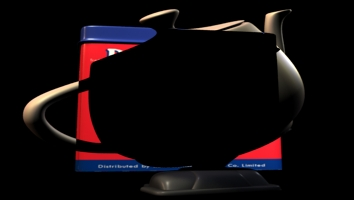``` fg := IceImage With("teapot.tif") bg := IceImage With("tinbox.tif") result := bg MergeXor(fg) ```
Out(fg)
fg: Foreground image (Image)

Merge out operation.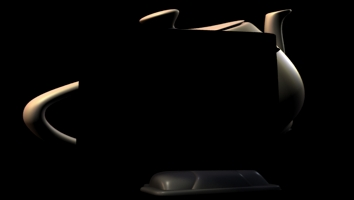``` fg := IceImage With("teapot.tif") bg := IceImage With("tinbox.tif") result := bg Out(fg) ```
TransmissionFilter(fg)
fg: Foreground image (Image)

In this operation, the foreground acts as a color filter for the background.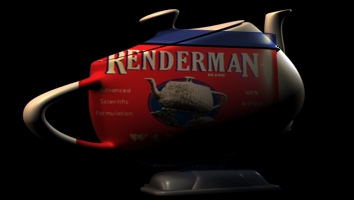``` fg := IceImage With("teapot.tif") bg := IceImage With("tinbox.tif") result := bg TransmissionFilter(fg) ```